# OpenMP topic: Loop parallelism

##### Experimental html version of Parallel Programming in MPI, OpenMP, and PETSc by Victor Eijkhout. download the textbook at https:/theartofhpc.com/pcse

$\newcommand\inv{^{-1}}\newcommand\invt{^{-t}} \newcommand\bbP{\mathbb{P}} \newcommand\bbR{\mathbb{R}} \newcommand\defined{ \mathrel{\lower 5pt \hbox{{\equiv\atop\mathrm{D}}}}}$ 19.1 : Loop parallelism
19.1.1 : Loops are static
19.1.2 : Exercises
19.2 : An example
19.3 : Loop schedules
19.4 : Reductions
19.5 : Nested loops
19.5.1 : Collapsing nested loops
19.5.2 : Array traversal
19.6 : Ordered iterations
19.7 : nowait
19.8 : While loops
19.9 : Review questions

# 19 OpenMP topic: Loop parallelism

## 19.1 Loop parallelism

crumb trail: > omp-loop > Loop parallelism

Loop parallelism is a very common type of parallelism in scientific codes, so OpenMP has an easy mechanism for it. OpenMP parallel loops are a first example of OpenMP worksharing' constructs (see section  21.1 for the full list): constructs that take an amount of work and distribute it over the available threads in a parallel region, created with the parallel pragma.

The parallel execution of a loop can be handled a number of different ways. For instance, you can create a parallel region around the loop, and adjust the loop bounds:

#pragma omp parallel
{
for (int i=low; i<high; i++)
// do something with i
}

In effect, this is how you would parallelize a loop in MPI: the parallel pragma creates a team of threads, each thread executes the block of code, and based on its thread number finds a unique block of work to do.

Exercise What is an important difference between the resulting OpenMP and MPI code?
End of exercise

A more natural option is to use the for pragma where OpenMP does the above chopping of the loop for you:

#pragma omp parallel
#pragma omp for
for (int i=0; i<N; i++) {
// do something with i
}

(In this example the loop variable is declared in the loop header, as is the preferred practice, but if you don't do it this way, the loop variable is automatically made private to the threads.)

Leaving the work distribution to OpenMP has several advantages. For one, you don't have to calculate the loop bounds for the threads yourself, but you can also tell OpenMP to assign the loop iterations according to different schedules (section  19.3  ).

Fortran note The for pragma only exists in C; there is a correspondingly named do pragma in Fortran.

$!omp parallel$!omp do
do i=1,N
! something with i
end do
$!omp end do$!omp end parallel

End of Fortran note

Figure  19.1 shows the execution on four threads of

#pragma omp parallel
{
code1();
#pragma omp for
for (int i=1; i<=4*N; i++) {
code2();
}
code3();
}

The code before and after the loop is executed identically in each thread; the loop iterations are spread over the four threads.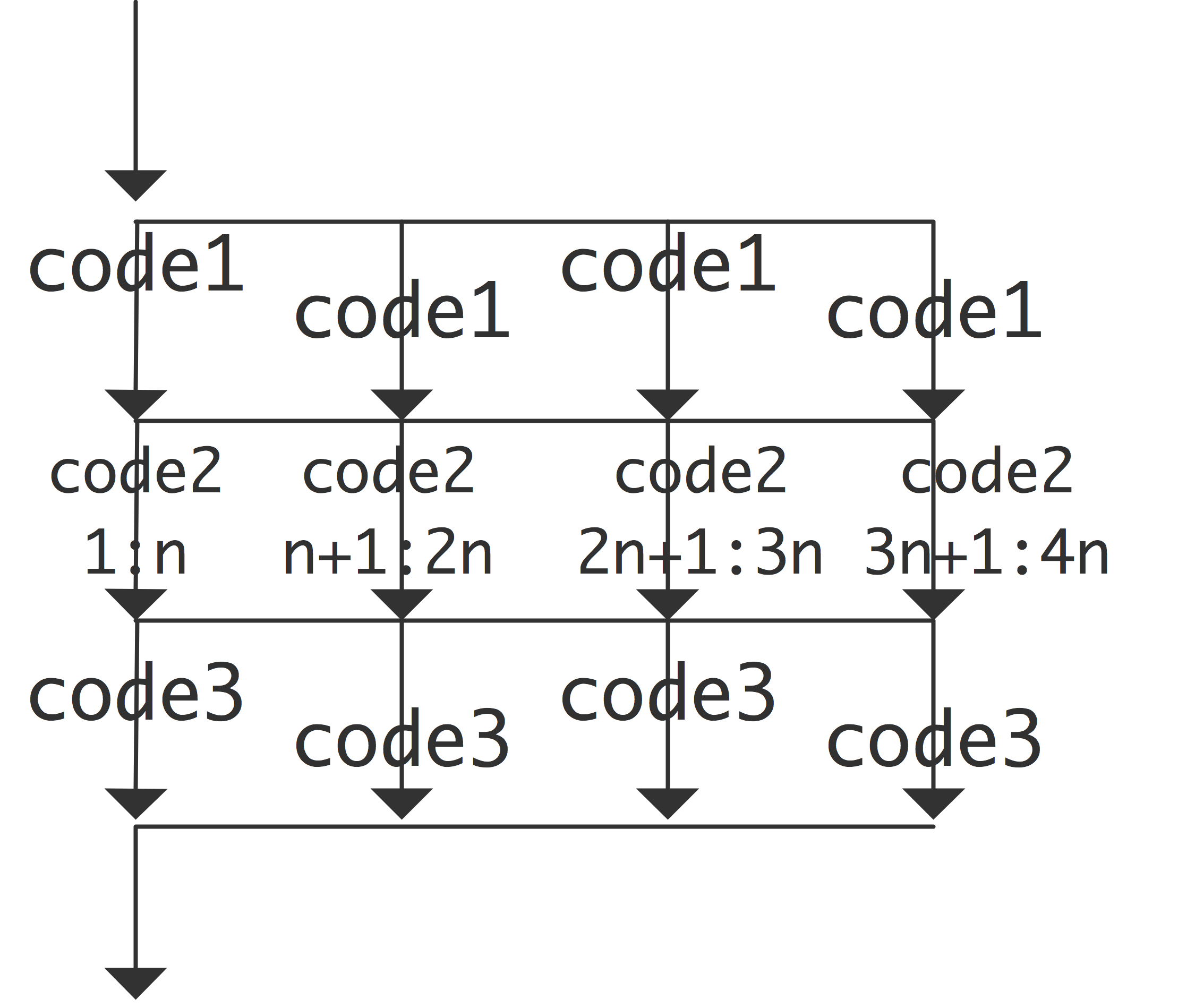FIGURE 19.1: Execution of parallel code inside and outside a loop

Note that the do and for pragmas do not create a team of threads: they take the team of threads that is active, and divide the loop iterations over them. This means that the omp for or omp do directive needs to be inside a parallel region.

Exercise What would happen in the above example if you increase the number of threads to be larger than the number of cores?
End of exercise

It is also possible to have a combined omp parallel for or omp parallel do directive.

#pragma omp parallel for
for (int i=0; .....


C++ note OpenMP can parallelize any range-based loop with a random-access iterator.

// iterator.cxx
class NewVector {
public:
// iterator stuff
class iter;
iter begin();
iter end();

};

The following methods are needed for the contained iter class:
NewVector::iter& operator++();
int& operator*();
bool operator==( const NewVector::iter &other ) const;
bool operator!=( const NewVector::iter &other ) const;
// needed to OpenMP
int operator-( const NewVector::iter& other ) const;

And then a range-based loop is allowed:
NewVector v(s);
#pragma omp parallel for
for ( auto e : v )
cout << e << " ";

End of C++ note

### 19.1.1 Loops are static

crumb trail: > omp-loop > Loop parallelism > Loops are static

There are some restrictions on the loop: basically, OpenMP needs to be able to determine in advance how many iterations there will be.

• The loop can not contains break  , return  , exit statements, or goto to a label outside the loop.
• The continue (for C/C++) or cycle (for Fortran) statement is allowed.
• The index update has to be an increment (or decrement) by a fixed amount.
• The loop index variable is automatically private (section  22.2  ), and no changes to it inside the loop are allowed. The following loop is not parallelizable in OpenMP:
      for (int i=0; i<N; ) {
// something
if (something)
i++;
else
i += 2;
}


Remark The loop index needs to be an integer value for the loop to be parallelizable. Unsigned values are allowed as of OpenMP-.
End of remark

### 19.1.2 Exercises

crumb trail: > omp-loop > Loop parallelism > Exercises

Exercise Compute $\pi$ by numerical integration  . We use the fact that $\pi$ is the area of the unit circle, and we approximate this by computing the area of a quarter circle using Riemann sums  .

• Let $f(x)=\sqrt{1-x^2}$ be the function that describes the quarter circle for $x=0\ldots 1$;
• Then we compute \begin{equation} \pi/4\approx\sum_{i=0}^{N-1} \Delta x f(x_i) \qquad \hbox{where $x_i=i\Delta x$ and $\Delta x=1/N$} \end{equation}
Write a program for this, and parallelize it using OpenMP parallel for directives.
1. Put a parallel directive around your loop. Does it still compute the right result? Does the time go down with the number of threads? (The answers should be no and no.)
2. Change the parallel to parallel for (or parallel do  ). Now is the result correct? Does execution speed up? (The answers should now be no and yes.)
3. Put a critical directive in front of the update. (Yes and very much no.)
4. Remove the critical and add a clause reduction (+:quarterpi) to the for directive. Now it should be correct and efficient.
Use different numbers of cores and compute the speedup you attain over the sequential computation. Is there a performance difference between the OpenMP code with 1 thread and the sequential code?
End of exercise

Remark In this exercise you may have seen the runtime go up a couple of times where you weren't expecting it. The issue here is \indexterm{false sharing}; see  Eijkhout:IntroHPC for more explanation.
End of remark

## 19.2 An example

crumb trail: > omp-loop > An example

To illustrate the speedup of perfectly parallel calculations, we consider a simple code that applies the same calculation to each element of an array.

All tests are done on the TACC Frontera cluster, which has dual-socket Intel Cascade Lake nodes, with a total of 56 cores. We control affinity by setting OMP_PROC_BIND=true  .

Here is the essential code fragment:

// speedup.c
#pragma omp parallel for
for (int ip=0; ip<N; ip++) {
for (int jp=0; jp<M; jp++) {
double f = sin( values[ip] );
values[ip] = f;
}
}


Exercise Verify that the outer loop is parallel, but the inner one is not.
End of exercise

Exercise Compare the time for the sequential code and the single-threaded OpenMP code. Try different optimization levels, and different compilers if you have them.

• Do you sometimes get a significant difference? What would be an explanation?
• Does your compiler have a facility for generating optimization reports? For instance -qoptreport=5 for the Intel compiler  .

End of exercise

Now we investigate the influence of two parameters:

1. the OpenMP thread count: while we have 56 cores, values larger than that are allowed; and
2. the size of the problem: the smaller the problem, the larger the relative overhead of creating and synchronizing the team of threads.
We execute the above computation several times to even out effects of cache loading.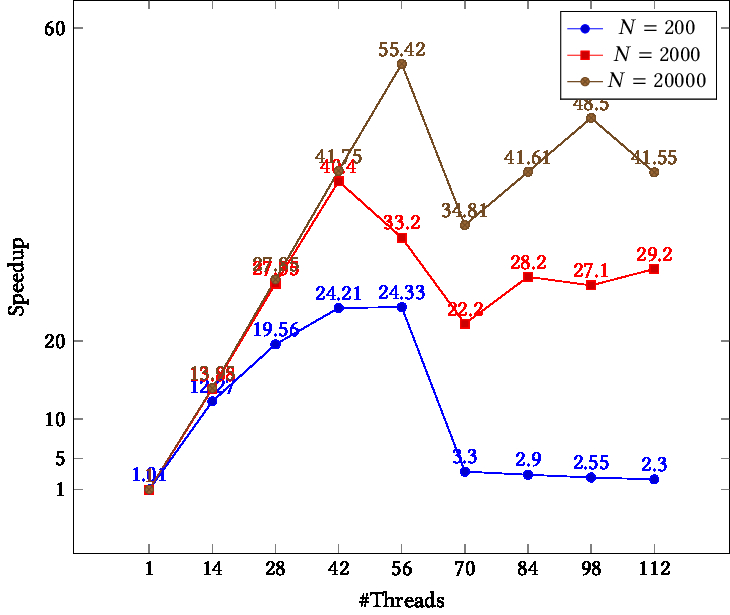TIKZPICTURE 19.2: Speedup as function of problem size

The results are in figure  19.2 :

• While the problem size is always larger than the number of threads, only for the largest problem, which has at least 400 points per thread, is the speedup essentially linear.
• OpenMP allows for the number of threads to be larger than the core count, but there is no performance improvement in doing so.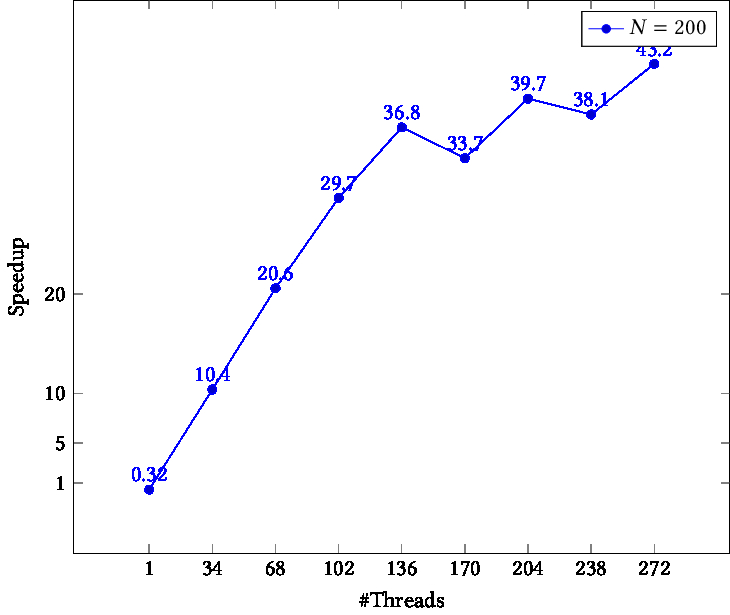TIKZPICTURE 19.3: Speedup on a hyper-threaded architecture

The above tests did not use hyperthread s, since that is disabled on Frontera. However, the Intel Knights Landing nodes of the TACC Stampede2 cluster have four hyperthread s per core. Table  19.3 shows that this will indeed give a modest speedup.

For reference, the commandlines executed were:

# frontera
make localclean run_speedup EXTRA_OPTIONS=-DN=200 NDIV=8 NP=112
make localclean run_speedup EXTRA_OPTIONS=-DN=2000 NDIV=8 NP=112
make localclean run_speedup EXTRA_OPTIONS=-DN=20000 NDIV=8 NP=112
# stampede2
make localclean run_speedup NDIV=8 EXTRA_OPTIONS="-DN=200000 -DM=1000" NP=272


C++ note Parallel loops in C++ can use range-based syntax as of OpenMP-:

// vecdata.cxx
#pragma omp parallel for
for ( auto& elt : values ) {
elt = 5.f;
}
float sum{0.f};
#pragma omp parallel for reduction(+:sum)
for ( auto elt : values ) {
sum += elt;
}

Tests not reported here show exactly the same speedup as the C code. End of C++ note

C++ note The \cppstandard{20} ranges library is also supported:

// range.cxx
#   pragma omp parallel for reduction(+:count)
for ( auto e : data )
count += e;
#   pragma omp parallel for reduction(+:count)
for ( auto e : data
| std::ranges::views::drop(1) )
count += e;
#   pragma omp parallel for reduction(+:count)
for ( auto e : data
| std::ranges::views::transform
( []( auto e ) { return 2*e; } ) )
count += e;

End of C++ note

C++ note \lstinputlisting{code/omp/cxx/range-ls6.runout} End of C++ note

## 19.3 Loop schedules

crumb trail: > omp-loop > Loop schedules

Usually you will have many more iterations in a loop than there are threads. Thus, there are several ways you can assign your loop iterations to the threads. OpenMP lets you specify this with the schedule clause.

#pragma omp for schedule(....)


The first distinction we now have to make is between static and dynamic schedules. With static schedules, the iterations are assigned purely based on the number of iterations and the number of threads (and the chunk parameter; see later). In dynamic schedules, on the other hand, iterations are assigned to threads that are unoccupied. Dynamic schedules are a good idea if iterations take an unpredictable amount of time, so that load balancing is needed.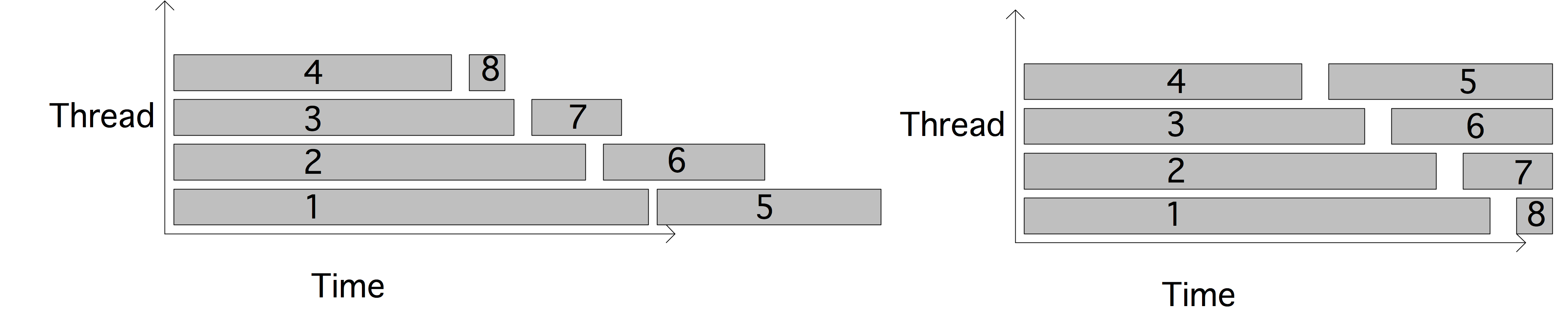FIGURE 19.4: Illustration static round-robin scheduling versus dynamic

Figure  19.4 illustrates this: assume that each core gets assigned two (blocks of) iterations and these blocks take gradually less and less time. You see from the left picture that thread 1 gets two fairly long blocks, where as thread 4 gets two short blocks, thus finishing much earlier. (This phenomenon of threads having unequal amounts of work is known as load imbalance  .) On the other hand, in the right figure thread 4 gets block 5, since it finishes the first set of blocks early. The effect is a perfect load balancing.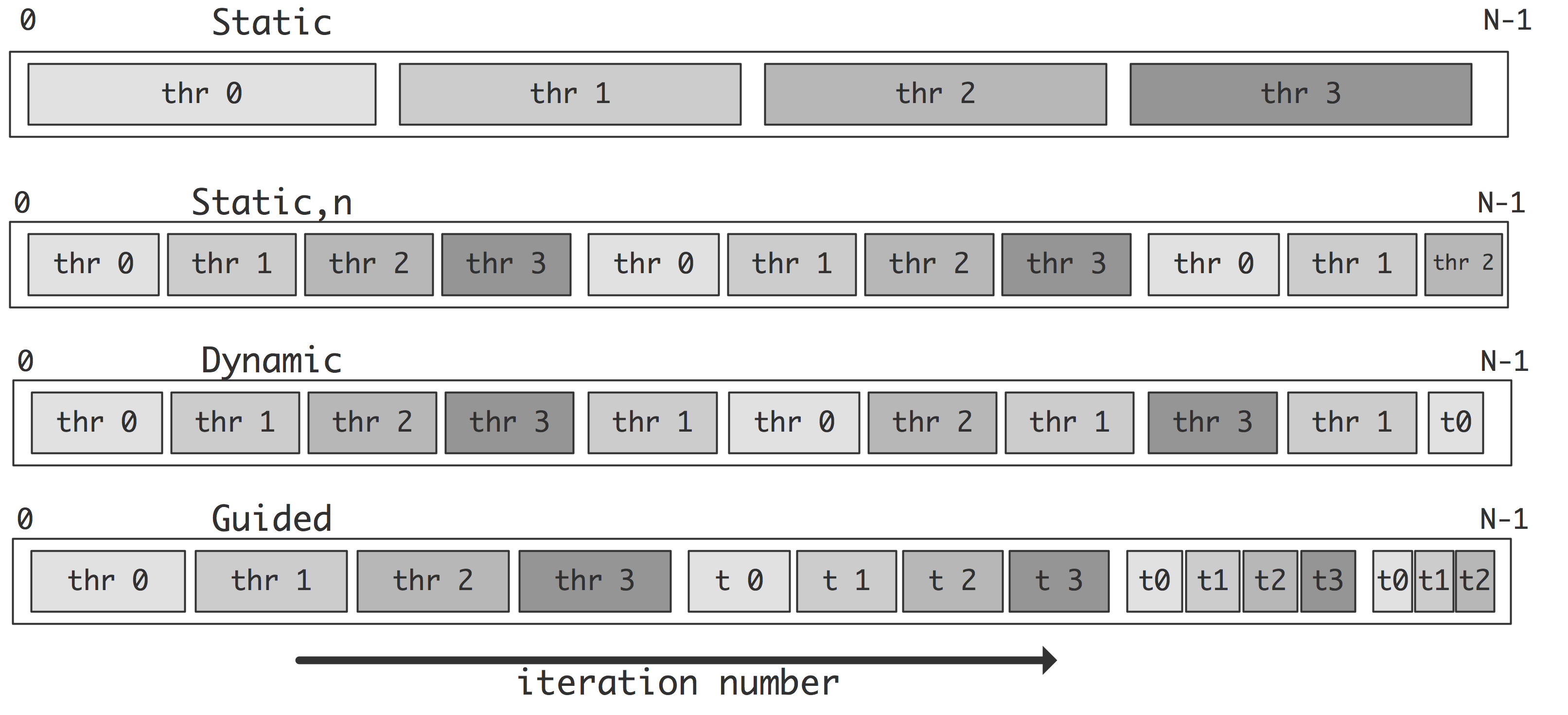FIGURE 19.5: Illustration of the scheduling strategies of loop iterations

The default static schedule is to assign one consecutive block of iterations to each thread. If you want different sized blocks you can define a \indexclauseoption{schedule}{chunk} size:

#pragma omp for schedule(static[,chunk])

(where the square brackets indicate an optional argument). With static scheduling, the compiler will determine the assignment of loop iterations to the threads at compile time, so, provided the iterations take roughly the same amount of time, this is the most efficient at runtime.

The choice of a chunk size is often a balance between the low overhead of having only a few chunks, versus the load balancing effect of having smaller chunks.

Exercise Why is a chunk size of 1 typically a bad idea? (Hint: think about cache lines, and read Eijkhout:IntroHPC  .)
End of exercise

In dynamic scheduling OpenMP will put blocks of iterations (the default chunk size is 1) in a task queue, and the threads take one of these tasks whenever they are finished with the previous.

#pragma omp for schedule(static[,chunk])

While this schedule may give good load balancing if the iterations take very differing amounts of time to execute, it does carry runtime overhead for managing the queue of iteration tasks.

Finally, there is the \indexclauseoption{schedule}{guided} schedule, which gradually decreases the chunk size. The thinking here is that large chunks carry the least overhead, but smaller chunks are better for load balancing. The various schedules are illustrated in figure  19.5  .

If you don't want to decide on a schedule in your code, you can specify the \indexclauseoption{schedule}{runtime} schedule. The actual schedule will then at runtime be read from the OMP_SCHEDULE environment variable. You can even just leave it to the runtime library by specifying \indexclauseoption{schedule}{auto}

Exercise We continue with exercise  19.1.2  . We add adaptive integration'% : where needed, the program refines the step size\footnote{It doesn't actually do this in a mathematically sophisticated way, so this code is more for the sake of the example.}. This means that the iterations no longer take a predictable amount of time.

for (int i=0; i<nsteps; i++) {
double
x = i*h,x2 = (i+1)*h,
y = sqrt(1-x*x),
y2 = sqrt(1-x2*x2),
slope = (y-y2)/h;
if (slope>15) slope = 15;
int
samples = 1+(int)slope, is;
for (int is=0; is<samples; is++) {
double
hs = h/samples,
xs = x+ is*hs,
ys = sqrt(1-xs*xs);
quarterpi += hs*ys;
nsamples++;
}
}
pi = 4*quarterpi;


1. Use the omp parallel for construct to parallelize the loop. As in the previous lab, you may at first see an incorrect result. Use the reduction clause to fix this.
2. Your code should now see a decent speedup, but possible not for all cores. It is possible to get completely linear speedup by adjusting the schedule.

Start by using schedule (static,n)  . Experiment with values for $n$. When can you get a better speedup? Explain this.

3. Since this code is somewhat dynamic, try schedule (dynamic)  . This will actually give a fairly bad result. Why? Use schedule (dynamic,$n$) instead, and experiment with values for $n$.
4. Finally, use schedule (guided)  , where OpenMP uses a heuristic. What results does that give?

End of exercise

Exercise Program the LU factorization algorithm without pivoting.

for k=1,n:
A[k,k] = 1./A[k,k]
for i=k+1,n:
A[i,k] = A[i,k]/A[k,k]
for j=k+1,n:
A[i,j] = A[i,j] - A[i,k]*A[k,j]

1. Argue that it is not possible to parallelize the outer loop.
2. Argue that it is possible to parallelize both the $i$ and $j$ loops.
3. Parallelize the algorithm by focusing on the $i$ loop. Why is the algorithm as given here best for a matrix on row-storage? What would you do if the matrix was on column storage?
4. Argue that with the default schedule, if a row is updated by one thread in one iteration, it may very well be updated by another thread in another. Can you find a way to schedule loop iterations so that this does not happen? What practical reason is there for doing so?

End of exercise

The schedule can be declared explicitly, set at runtime through the OMP_SCHEDULE environment variable, or left up to the runtime system by specifying auto  . Especially in the last two cases you may want to enquire what schedule is currently being used with omp_get_schedule  .

int omp_get_schedule(omp_sched_t * kind, int * modifier );


Its mirror call is omp_set_schedule  , which sets the value that is used when schedule value runtime is used. It is in effect equivalent to setting the environment variable OMP_SCHEDULE  .

void omp_set_schedule (omp_sched_t kind, int modifier);


 \toprule Type environment variable clause omp_sched_t omp_sched_t modifier default {\tt OMP\_SCHEDULE\char\=} {\tt schedule( ... )} name value \midrule static static[,n] {static[,n]} omp_sched_static 1 $N/\mathit{nthreads}$ dynamic dynamic[,n] {dynamic[,n]} omp_sched_dynamic 2 $1$ guided guided[,n] {guided[,n]} omp_sched_guided 3 auto auto auto omp_sched_auto 4 \bottomrule

Here are the various schedules you can set with the schedule clause:

• [affinity] Set by using value omp_sched_affinity
• [auto] The schedule is left up to the implementation. Set by using value omp_sched_auto
• [static] value: 1. The modifier parameter is the chunk size. Can also be set by using value omp_sched_static
• [dynamic] value: 2. The modifier parameter is the chunk size; default 1. Can also be set by using value omp_sched_dynamic
• [guided] Value: 3. The modifier parameter is the chunk size. Set by using value omp_sched_guided
• [runtime] Use the value of the OMP_SCHEDULE environment variable. Set by using value omp_sched_runtime

## 19.4 Reductions

crumb trail: > omp-loop > Reductions

So far we have focused on loops with independent iterations. Reductions are a common type of loop with dependencies. There is an extended discussion of reductions in chapter  OpenMP topic: Loop parallelism  .

## 19.5 Nested loops

crumb trail: > omp-loop > Nested loops

### 19.5.1 Collapsing nested loops

crumb trail: > omp-loop > Nested loops > Collapsing nested loops

In general, the more work there is to divide over a number of threads, the more efficient the parallelization will be. In the context of parallel loops, it is possible to increase the amount of work by parallelizing all levels of loops instead of just the outer one.

Example: in

for ( int i=0; i<N; i++ )
for ( int j=0; j<N; j++ )
A[i][j] = B[i][j] + C[i][j]

all $N^2$ iterations are independent, but a regular omp for directive will only parallelize one level. The \indexclause{collapse} clause will parallelize more than one level:
#pragma omp for collapse(2)
for ( int i=0; i<N; i++ )
for ( int j=0; j<N; j++ )
A[i][j] = B[i][j] + C[i][j]

It is only possible to collapse perfectly nested loops, that is, the loop body of the outer loop can consist only of the inner loop; there can be no statements before or after the inner loop in the loop body of the outer loop. That is, the two loops in
for ( int i=0; i<N; i++ ) {
y[i] = 0.;
for ( int j=0; j<N; j++)
y[i] += A[i][j] * x[j]
}

can not be collapsed.

Exercise You could rewrite the above code as

for (int i=0; i<N; i++)
y[i] = 0.;
for (int i=0; i<N; i++) {
for (int j=0; j<N; j++)
y[i] += A[i][j] * x[j]
}

Is it now correct to have the collapse directive on the nested loop?
End of exercise

Exercise Consider this code for matrix transposition:

void transposer(int n, int m, double *dst, const double *src) {
int blocksize;
for (int i = 0; i < n; i += blocksize) {
for (int j = 0; j < m; j += blocksize) {
// transpose the block beginning at [i,j]
for (int k = i; k < i + blocksize; ++k) {
for (int l = j; l < j + blocksize; ++l) {
dst[k + l*n] = src[l + k*m];
}
}
}
}
}

Assuming that the src and dst array are disjoint, which loops are parallel, and how many levels can you collapse?
End of exercise

### 19.5.2 Array traversal

crumb trail: > omp-loop > Nested loops > Array traversal

Consider arrays

  float Amat[N][N];
float xvec[N],yvec[N];

and the operation $s\leftarrow y^tAx$.

1. Code this as an OpenMP parallel double loop.
2. Argue that the matrix $A$ can be traversed two ways: by rows and columns, or by columns and rows, both giving the same result (in exact arithmetic).
3. Argue that the loops can be collapsed with collapse directory.
4. So now you have 4 variants in addition to the sequential code. Time these.

You should find that the row/column (or row-major  ) variant is faster. Can you find reasons for this?

affinity  . 25.4  .

## 19.6 Ordered iterations

crumb trail: > omp-loop > Ordered iterations

Iterations in a parallel loop that are executed in parallel do not execute in lockstep. That means that in

#pragma omp parallel for
for ( ... i ... ) {
... f(i) ...
printf("something with %d\n",i);
}

it is not true that all function evaluations happen more or less at the same time, followed by all print statements. The print statements can really happen in any order. The \indexclause{ordered} clause coupled with the ordered directive can force execution in the right order:
#pragma omp parallel for ordered
for ( ... i ... ) {
... f(i) ...
#pragma omp ordered
printf("something with %d\n",i);
}

Example code structure:
#pragma omp parallel for shared(y) ordered
for ( ... i ... ) {
int x = f(i)
#pragma omp ordered
y[i] += f(x)
z[i] = g(y[i])
}

There is a limitation: each iteration can encounter only one ordered directive.

## 19.7nowait

crumb trail: > omp-loop > nowait

An OpenMP loop is a worksharing construct, after which execution in the parallel region goes back to replicated execution. To synchronize this, OpenMP inserts a barrier  , meaning that threads wait for each other to reach this point. See section  23.1.1 for details.

The implicit barrier at the end of a work sharing construct can be cancelled with a \indexclause{nowait} \index[omp]{barrier!cancelled by nowait} clause. This has the effect that threads that are finished can continue with the next code in the parallel region:

#pragma omp parallel
{
#pragma omp for nowait
for (int i=0; i<N; i++) {
...
}
// more parallel code
}


In the following example, threads that are finished with the first loop can start on the second. Note that this requires both loops to have the same schedule. We specify the static schedule here to have an identical scheduling of iterations over threads:

#pragma omp parallel
{
x = local_computation()
#pragma omp for schedule(static) nowait
for (int i=0; i<N; i++) {
x[i] = ...
}
#pragma omp for schedule(static)
for (int i=0; i<N; i++) {
y[i] = ... x[i] ...
}
}


## 19.8 While loops

crumb trail: > omp-loop > While loops

OpenMP can only handle for' loops: while loops can not be parallelized. So you have to find a way around that. While loops are for instance used to search through data:

while ( a[i]!=0 && i<imax ) {
i++; }
// now i is the first index for which
<tt>a[i]</tt>
is zero.

We replace the while loop by a for loop that examines all locations:
result = -1;
#pragma omp parallel for
for (int i=0; i<imax; i++) {
if (a[i]!=0 && result<0) result = i;
}


Exercise Show that this code has a race condition.
End of exercise

You can fix the race condition by making the condition into a critical section; section  23.2.2  . In this particular example, with a very small amount of work per iteration, that is likely to be inefficient in this case (why?). A more efficient solution uses the lastprivate pragma:

result = -1;
#pragma omp parallel for lastprivate(result)
for (int i=0; i<imax; i++) {
if (a[i]!=0) result = i;
}

You have now solved a slightly different problem: the result variable contains the last location where a[i] is zero.

## 19.9 Review questions

crumb trail: > omp-loop > Review questions

Exercise The following loop can be parallelized with a parallel for . Is it correct to add the directive collapse(2) ?

for (int i=0; i<N; i++) {
y[i] = 0.;
for (int j=0; j<N; j++)
y[i] += A[i][j] * x[j]
}


End of exercise

Exercise Same question for the nested loop here:

for (int i=0; i<N; i++)
y[i] = 0.;
for (int i=0; i<N; i++) {
for (int j=0; j<N; j++)
y[i] += A[i][j] * x[j]
}


End of exercise

Exercise In this triple loop:

for (int i=0; i<n; i++)
for (int j=0; j<n; j++)
for (int k=0; k<kmax; k++)
x[i][j] += f(i,j,k)

what OpenMP directives do you use? Can you collapse all levels? Does it matter what the loop bounds are?
End of exercise